# Introduction

The cell-key method (CKM) based random noise approach is a post-tabular Statistical Disclosure Control (SDC) technique. Random noise will be added to each table cell, according to some noise probability distribution - also referred to as perturbation table - and a mechanism to draw from that noise distribution in the so-called lookup step. The perturbation table consists of transition probabilities that describe the probabilities of transitioning from one state (e.g. a given original frequency count) to another (e.g. target frequency count). In other words, they are referred to as the probabilities of the random noises.

The goal of this package is to produce perturbation tables that can be used for applying random noise to statistical tables (also described as “to perturb statistical tables”) by SDC tools like the R-package cellKey or the software Tau-Argus.

## Features of the package

We now briefly mention the features of the ptable package before we actually show how to create perturbation tables in the following sections.

### Main features

As suggested in Marley and Leaver (2011) and illustrated in Giessing (2016) the package implements a maximum entropy approach to compute transition probabilities that guarantee certain characteristics for the random noise:

• The maximum noise (i.e. absolute value of any perturbation) is less than a specified integer value D.

• One of the main characteristics is the zero mean or unbiased noise in the sense that the expected bias of each separate original frequency is 0. This important characteristic guarantees unbiased noise independent of the distribution of original frequencies in a hypercube or set of hypercubes.

• The perturbations have a fixed noise variance $$\sigma ^2$$ that determines the spread of the possible noise values.

• The perturbations will produce no negative frequency counts.

• In the perturbed table will be no positive frequency counts less than a specified threshold value js.

### Graphical User interface (GUI)

In the ptable package there is also a shiny app for first time users and visual-style learners. The shiny app provides a visualization mode using the function ptable() and allows users to experiment with different parameter settings while getting direct feedback by means of graphical plots and summaries. In this way, users can visually learn how parameters effect the probability distribution.

Note: The current GUI provides perturbation tables for frequency tables (table = 'cnts') only.

### Auxiliary features

The package provides auxiliary functions that allow to process and review the results. Besides the visualization mode of the shiny app, users have also a code-based possibility to create visualization plots directly using the function plot(). The function allows to display a variety of plots (among others the noise probability distribution plot, a perturbation panel, etc.).

Further, pt_export() allows users to write the perturbation table into an external file. The format of the file can be specified such that it can be made available to Tau-Argus or the software SAS.

# Frequency Tables

Note: The following examples are illustrated for frequency tables with cell counts. Differences to magnitude tables with continuous/numeric variables are highlighted in the subsequent section.

## Examples of the main features

library(ptable)
packageVersion("ptable")
#>  '1.0.0'

### Minimal Parameter Setting and Computation of the Transition Probabilities

#### Computation of the Transition Probabilities

# note:
# all parameters except for maximum noise D and variance V have default values
ptab <- create_cnt_ptable(D = 2, V = 1)

The minimum set of parameters that have to be specified are:

• D: the maximum noise/perturbation (a positive integer) and
• V: the noise or perturbation variance (a positive integer).

The result of the above function is an object of class “ptable” which contains the following slots:

str(ptab)
#> Formal class 'ptable' [package "ptable"] with 8 slots
#>   ..@ tMatrix   : num [1:3, 1:5] 1 0.3665 0.0638 0 0.3665 ...
#>   .. ..- attr(*, "dimnames")=List of 2
#>   .. .. ..$: chr [1:3] "0" "1" "2" #> .. .. ..$ : chr [1:5] "0" "1" "2" "3" ...
#>   ..@ pClasses  : num [1:3] 0 1 2
#>   ..@ pTable    :Classes 'data.table' and 'data.frame':  10 obs. of  7 variables:
#>   .. ..$i : num [1:10] 0 1 1 1 1 2 2 2 2 2 #> .. ..$ j       : num [1:10] 0 0 1 2 3 0 1 2 3 4
#>   .. ..$p : num [1:10] 1 0.3665 0.3665 0.1676 0.0995 ... #> .. ..$ v       : num [1:10] 0 -1 0 1 2 -2 -1 0 1 2
#>   .. ..$p_int_lb: num [1:10] 0 0 0.366 0.733 0.901 ... #> .. ..$ p_int_ub: num [1:10] 1 0.366 0.733 0.901 1 ...
#>   .. ..$type : chr [1:10] "all" "all" "all" "all" ... #> .. ..- attr(*, ".internal.selfref")=<externalptr> #> .. ..- attr(*, "intervals")= chr "default" #> ..@ empResults:Classes 'data.table' and 'data.frame': 3 obs. of 6 variables: #> .. ..$ i     : num [1:3] 0 1 2
#>   .. ..$p_mean: num [1:3] 0 0 0 #> .. ..$ p_var : num [1:3] 0 0.932 1
#>   .. ..$p_sum : num [1:3] 1 1 1 #> .. ..$ p_stay: num [1:3] 1 0.366 0.383
#>   .. ..\$ iter  : int [1:3] 0 20 1
#>   .. ..- attr(*, ".internal.selfref")=<externalptr>
#>   .. ..- attr(*, "sorted")= chr "i"
#>   ..@ pParams   :Formal class 'ptable_params' [package "ptable"] with 12 slots
#>   .. .. ..@ D    : int 2
#>   .. .. ..@ V    : num 1
#>   .. .. ..@ js   : int 0
#>   .. .. ..@ ncat : int 2
#>   .. .. ..@ pstay: num [1:2] NA NA
#>   .. .. ..@ optim: int [1:2] 1 1
#>   .. .. ..@ mono : logi [1:2] TRUE TRUE
#>   .. .. ..@ table: chr "cnts"
#>   .. .. ..@ icat : int [1:2] 1 2
#>   .. .. ..@ step : int 1
#>   .. .. ..@ type : chr "all"
#>   .. .. ..@ label: chr "D2V100"
#>   ..@ tStamp    : chr "20230301164637"
#>   ..@ type      : chr "all"
#>   ..@ table     : chr "cnts"

The most relevant and important slots of the object are:

• tMatrix: A transition matrix that describes the perturbation probabilities from one state (original frequency count) to another (target frequency count) .
• pTable: Data table needed for the lookup step of a SDC tool that can apply random noise to statistical tables (e.g. the cellKey package or the software Tau-Argus). However, in the following sections there will be explained, how the tables can be used or exported by auxiliary functions.
• pParams: The input parameters that result from the preceding function pt_create_pParams().
• empResults: A data frame for output checking of the constraints.

#### The Transition Matrix

Let’s have a look at the transition matrix (i.e. at the slot @tMatrix) of the object ptab:

# note: to look at a specific slot, just name the object and add the
# corresponding slot with a leading "@"
ptab@tMatrix
#>            0         1         2          3          4
#> 0 1.00000000 0.0000000 0.0000000 0.00000000 0.00000000
#> 1 0.36648551 0.3664855 0.1675725 0.09945652 0.00000000
#> 2 0.06382714 0.2446915 0.3829628 0.24469145 0.06382714

Each row of the transition matrix represents the noise distribution for an original frequency count. The probability that an original frequency count of 1 becomes a 3 (i.e. the 1 is perturbed with a noise value of +2) is 0.0994565.

diag(ptab@tMatrix)
#>         0         1         2
#> 1.0000000 0.3664855 0.3829628

The main diagonal shows the preservation probabilities. These are the probabilities that the original frequencies remain unchanged. In this instance, the probability that an original frequency 2 remains unchanged is 0.3829628.

#### Symmetry - and what does it mean in the context of perturbation tables?

As you may have recognized, the transition matrix has a finite number of rows (that represent original frequency counts) and columns (that represent the target frequency counts).

# let's have a look at the number of different original positive frequency
# counts that will be treated
params <- slot(ptab, "pParams")
params@ncat
#>  2
# if this number is added by +1 (for the zero count) we get
params@ncat + 1
#>  3

The number depends on both, the maximum perturbation D and the threshold value js. The last row of the transition matrix delivers a symmetric distribution. This distribution will be applied to each original frequency larger than this frequency.

# the object @pClasses shows all original frequencies
# that have their own distribution
ptab@pClasses
#>  0 1 2

# symmetry is achieved for the original frequency count i=...
max(ptab@pClasses)
#>  2
# or
ptab@pClasses[params@ncat + 1]
#>  2

In the given example, each frequency count larger than 2 will be perturbed according to the distribution for i=2. For example, in case of i=3 the distribution reads as follows

#>          1          2          3          4          5
#> 0.06382714 0.24469145 0.38296282 0.24469145 0.06382714

or in case of i=12326

#>      12324      12325      12326      12327      12328
#> 0.06382714 0.24469145 0.38296282 0.24469145 0.06382714

Given this symmetry, the transition matrix can be displayed in the reduced form. There is no need to define more rows than up to the case of symmetry.

#### Output Checking and Troubleshooting

Next, we will check the empirical results that can be used for troubleshooting:

ptab@empResults
#>    i p_mean   p_var p_sum  p_stay iter
#> 1: 0      0 0.00000     1 1.00000    0
#> 2: 1      0 0.93188     1 0.36649   20
#> 3: 2      0 1.00000     1 0.38296    1

The matrix has the following columns:

• i: indicates the original frequency to which the remaining columns are referred to
• p_mean: shows the bias of the perturbation of an original frequency (should be zero: unbiasedness)
• p_var: shows the noise variance of an original frequency (Note: If p_var differs from the chosen input parameter V - as it does in the example above - we have a violation of the fixed variance condition. In that case, we have to set a different variance parameter or to change other parameters!)
• p_sum: the sum of the transition probabilities for an original frequency count must equal to 1
• p_stay: corresponds to the diagonal of the transition matrix
• iter: any value other than 1 points out discrepancies (e.g. violation of the fixed variance parameter)1

As can be seen in the example, the preset variance of V=1 does not hold for the original frequency i=1. To fulfill the condition of a fixed variance, we re-run the computation with a different variance parameter. Let’s try a variance parameter V=0.9:

ptab_new <- create_cnt_ptable(D = 2, V = 0.9)
ptab_new@empResults
#>    i p_mean p_var p_sum  p_stay iter
#> 1: 0      0   0.0     1 1.00000    0
#> 2: 1      0   0.9     1 0.36250    1
#> 3: 2      0   0.9     1 0.40928    1

The new computation with the updated variance parameter results in a perturbation table that fulfills all conditions. Of course, the resulting transition matrix now differs from the first one:

ptab_new@tMatrix
#>            0         1         2         3          4
#> 0 1.00000000 0.0000000 0.0000000 0.0000000 0.00000000
#> 1 0.36250000 0.3625000 0.1875000 0.0875000 0.00000000
#> 2 0.05154609 0.2438156 0.4092765 0.2438156 0.05154609

### The default ptable and the modified ptable

#### The default ptable

For our SDC tools - the cellKey-package and TauArgus - we use the ptable with noise intervals with result after accumulating the noise probabilities

ptab_new@pTable
#>     i j          p  v   p_int_lb   p_int_ub type
#>  1: 0 0 1.00000000  0 0.00000000 1.00000000  all
#>  2: 1 0 0.36250000 -1 0.00000000 0.36250000  all
#>  3: 1 1 0.36250000  0 0.36250000 0.72500000  all
#>  4: 1 2 0.18750000  1 0.72500000 0.91250000  all
#>  5: 1 3 0.08750000  2 0.91250000 1.00000000  all
#>  6: 2 0 0.05154609 -2 0.00000000 0.05154609  all
#>  7: 2 1 0.24381564 -1 0.05154609 0.29536173  all
#>  8: 2 2 0.40927654  0 0.29536173 0.70463827  all
#>  9: 2 3 0.24381564  1 0.70463827 0.94845391  all
#> 10: 2 4 0.05154609  2 0.94845391 1.00000000  all

The noise intervals in the standard ptable are ordered from -D to D. A modified ptable still has the same properties as the standard ptable but can ensure a higher protection of perturbed frequency tables since the noise probabilities are split and the intervals are rearranged.

#### The modified ptable

Modify the ptable to have a higher level of protection

mod_ptab_new <-
modify_cnt_ptable(ptab_new, threshold = 0.2, seed = 123)
#> There are 1 consecutive intervals with identical noise. You can try another
#>           'seed' or proceed. At least, check whether the modified ptable has
#>           sufficiently rearranged sub-intervals.
#>
#>  NOTE for Tau-Argus:
#>           Please use a new Tau-Argus Release (>= 4.2.3).

The noise probabilities larger than the threshold value will be split. Then, the split noise probabilities are randomly rearranged using a seed. Finally, the intervals of the ptable will be adjusted.

mod_ptab_new@pTable
#>     i j          p  v  p_int_lb  p_int_ub type
#>  1: 0 0 1.00000000  0 0.0000000 1.0000000  all
#>  2: 1 3 0.08750000  2 0.0000000 0.0875000  all
#>  3: 1 1 0.20000000  0 0.0875000 0.2875000  all
#>  4: 1 0 0.20000000 -1 0.2875000 0.4875000  all
#>  5: 1 1 0.16250000  0 0.4875000 0.6500000  all
#>  6: 1 2 0.18750000  1 0.6500000 0.8375000  all
#>  7: 1 0 0.16250000 -1 0.8375000 1.0000000  all
#>  8: 2 2 0.20000000  0 0.0000000 0.2000000  all
#>  9: 2 2 0.00927654  0 0.2000000 0.2092765  all
#> 10: 2 3 0.20000000  1 0.2092765 0.4092765  all
#> 11: 2 1 0.20000000 -1 0.4092765 0.6092765  all
#> 12: 2 0 0.05154609 -2 0.6092765 0.6608226  all
#> 13: 2 1 0.04381564 -1 0.6608226 0.7046383  all
#> 14: 2 2 0.20000000  0 0.7046383 0.9046383  all
#> 15: 2 3 0.04381564  1 0.9046383 0.9484539  all
#> 16: 2 4 0.05154609  2 0.9484539 1.0000000  all

The goal of the following subsections is to create perturbation tables with extended and afterwards with advanced parameter settings of the function create_cnt_ptable().

### Extended Parameter Setting

The remaining parameters (among others js and pstay), are set to default if not specified:

• js: the perturbations will not produce target frequencies equal to or below this threshold value (a non-negative integer; default: js=0)
• pstay: the probability of an original frequency to remain unperturbed (a positive value; default: pstay=NA no preset probability; this default produces the maximum entropy solution)
• mono: monotony condition (logical; default: mono=TRUE to force monotony). The idea is to produce distributions where transition probabilities decrease monotonously when the distance of the target frequency to the original frequency increases.

However, let’s change and modify them with respect to our needs:

#### Setting the probability of an original frequency to remain unperturbed

# note:  once again, check the diagonal entries
ptable_e21 <- create_cnt_ptable(D = 4, V = 1, pstay = 0.5)
# note:  once again, check the empirical results or the diagonal entries
ptable_e21@empResults
#>    i p_mean p_var p_sum p_stay iter
#> 1: 0      0     0     1    1.0    0
#> 2: 1      0     1     1    0.5    1
#> 3: 2      0     1     1    0.5    1
#> 4: 3      0     1     1    0.5    1
#> 5: 4      0     1     1    0.5    1
diag(ptable_e21@tMatrix)
#>   0   1   2   3   4
#> 1.0 0.5 0.5 0.5 0.5

The probability of an original frequency to remain unperturbed (i.e. that the target frequency equals the original frequency) is set to 50%. In the advanced parameter settings we will learn how we can assign a specific parameter to each original frequency count.

Important note regarding “false positives”: Zeroes will not be perturbed to positive frequency counts since unbiasedness is a main characteristic of the cell-key based method.2

#### Do not allow for positive cell values equal to or below a specified threshold value

ptable_e22 <- create_cnt_ptable(D = 5, V = 3, js = 2)
ptable_e22@tMatrix
#>            0           3          4          5           6            7
#> 0 1.00000000 0.000000000 0.00000000 0.00000000 0.000000000 0.0000000000
#> 1 0.73446954 0.136720190 0.07279631 0.03742956 0.018584399 0.0000000000
#> 2 0.40880704 0.406643537 0.14762401 0.03231882 0.004266868 0.0003397188
#> 3 0.20398618 0.393662525 0.24749239 0.11056847 0.035102043 0.0079189055
#> 4 0.08485487 0.277921233 0.27792123 0.18939369 0.105125448 0.0454553570
#> 5 0.02014749 0.173347467 0.22063302 0.22131828 0.174967643 0.1090164652
#> 6 0.00000000 0.072029825 0.13671708 0.19735138 0.216652933 0.1808822561
#> 7 0.00000000 0.018439196 0.05548389 0.12162104 0.194208159 0.2259140226
#> 8 0.00000000 0.003791861 0.01659093 0.05229263 0.118730368 0.1941934608
#>             8           9          10          11          12         13
#> 0 0.000000000 0.000000000 0.000000000 0.000000000 0.000000000 0.00000000
#> 1 0.000000000 0.000000000 0.000000000 0.000000000 0.000000000 0.00000000
#> 2 0.000000000 0.000000000 0.000000000 0.000000000 0.000000000 0.00000000
#> 3 0.001269491 0.000000000 0.000000000 0.000000000 0.000000000 0.00000000
#> 4 0.015310774 0.004017391 0.000000000 0.000000000 0.000000000 0.00000000
#> 5 0.053532830 0.020717693 0.006319113 0.000000000 0.000000000 0.00000000
#> 6 0.114850887 0.055459983 0.020367237 0.005688418 0.000000000 0.00000000
#> 7 0.191441399 0.118180404 0.053146176 0.017410672 0.004155049 0.00000000
#> 8 0.228801496 0.194193460 0.118730371 0.052292634 0.016590928 0.00379186

Since the threshold value js is set to 2, we do not allow target frequencies 1 and 2. Hence, the corresponding columns contain zero probabilities.

Important note regarding “false zeroes”: Since the possibility of false zeroes is an important strength of a perturbative SDC method, zeroes are always allowed and cannot be excluded from the set of target frequencies.

Further parameters of the function create_cnt_ptable() are explained in the help page.

Several parameters of the function create_cnt_ptable() that we have learned so far, can also be assigned to each original positive frequency count separately using the vector notation in R.

First, we recommend to check how many different entries do we need. Therefore, let’s begin with the simple parameter setting

result <- create_cnt_ptable(D = 4, V = 1)
params <- slot(result, "pParams")
# and let's check the number of different positive original frequencies
params@ncat
#>  4

Let’s assume we want to assign different probabilities for pstay, one for each positive original count, we have to specify a vector of length 4:

# note: so far we have used a scalar for "pstay"", but now we use a vector
result <-
create_cnt_ptable(D = 4,
V = 1,
pstay = c(0.5, 0.5, 0.7, 0.8))
params <- slot(result, "pParams")

# let's check the results
result@empResults
#>    i p_mean p_var p_sum p_stay iter
#> 1: 0      0     0     1    1.0    0
#> 2: 1      0     1     1    0.5    1
#> 3: 2      0     1     1    0.5    1
#> 4: 3      0     1     1    0.7    1
#> 5: 4      0     1     1    0.8    1

The perturbation table we just created is arranged such that 50% of ones and twos, 70% of i=3 and 80% of all frequencies i>3 retain unperturbed.

The same holds for the argument mono.

## Examples of the auxiliary features

### Analyzing and plotting the results

# note: in the example we are working with the following result
result <-
create_cnt_ptable(
D = 2,
V = 1.08,
js = 1,
mono = c(TRUE, TRUE, FALSE, TRUE)
)

The resulting object result can now be plotted using the function plot(). According to the specification of the argument type, we will receive different plots as shown in the following subsections.

#### Plotting the Transition Probabilities in a Matrix

# note:  we have to use the argument type for specifying the plot
plot(result, type = "t")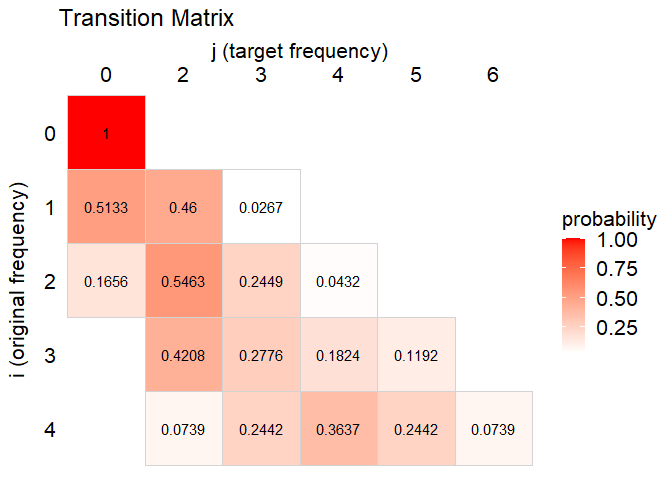The transition matrix plot is a heat-map plot indicating the probability (the darker the background of a transition probability, the higher the probability). Since the threshold value is set to js=1, we don’t allow for ones. That means, the target frequencies 1 are not allowed and, hence, the corresponding column in the transition matrix only consist of zero probabilities.

#### Plotting the Perturbation Panel

The perturbation table can be graphically represented as a perturbation panel:

plot(result, type = "p")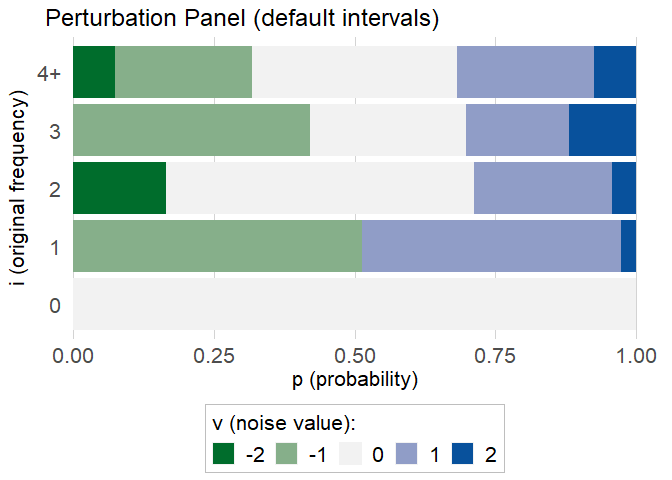Remember: For the demonstration we use a perturbation panel with default intervals.

Each bar corresponds to an original frequency count, different colors correspond to different noise values, and the width of the colored sub-bars corresponds to the respective transition probability.

An original frequency count i=1 changes with probability 0.5133 to j = 0. The adjustment equals -1. Accordingly a light blue bar of length 0.5133 is drawn in the panel. With a probability of 0.46 the original i = 1 becomes j = 2, so the perturbation equals +1. A light green bar of length 0.46 is attached. Finally, with a probability of 0.0267 the original i = 1 becomes j = 3, so the perturbation equals +2. Accordingly a dark green bar of length 0.0267 is attached. This way, all probabilities for the row i = 1 of the perturbation table are shown in the panel. The probabilities for i = 2, 3 and 4 are shown in the panel in a similar way.

It can be seen from the perturbation panel where the x-axis shown at the bottom with probability between 0 and 1 corresponds to a cumulative probability distribution.

#### Plotting the Distribution of the Perturbation Values/Noise

plot(result, type = "d")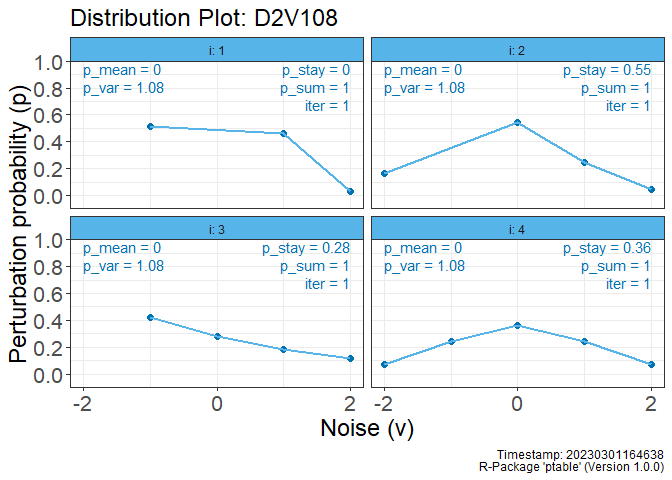The distribution plot of the perturbation/noise values is very helpful for designing the noise and troubleshooting. Each panel of the plot represents an original frequency count. The most important information of the empirical results (see section above) are also given within each panel: M shows the bias, V is the achieved variance (and not the preset variance parameter!). Further, violations are reported in red (e.g. in case of a not fulfilled fixed variance).

#### Save the plot to a file

The plots shown above can also be saved to a file (e.g. png, pdf):

plot(result, type = "d", file = "graph.pdf")

### Exporting the results

To use the perturbation table in SDC tools like Tau-Argus or SAS, users can export the result:

# for Tau-Argus
pt_export(result, file = "ptable.txt", SDCtool = "TauArgus")
# or for SAS
pt_export(result, file = "ptable.txt", SDCtool = "SAS")

Note: For the purpose of production please modify the ptable and don’t use the ptable with default intervals. But be aware of the interpretation using the perturbation panel.

## Troubleshooting Example: Violation of the fixed variance condition

Let’s assume we want to assign different probabilities for pstay as done in an example above, but with very large values:

result <-
create_cnt_ptable(D = 4,
V = 1.5,
pstay = c(0.8, 0.9, 0.9, 0.9))
# let's check the results
result@empResults
#>    i p_mean   p_var p_sum  p_stay iter
#> 1: 0      0 0.00000     1 1.00000    0
#> 2: 1      0 1.22497     1 0.38585   20
#> 3: 2      0 1.50000     1 0.60000    7
#> 4: 3      0 1.50000     1 0.70000    5
#> 5: 4      0 1.50000     1 0.80000    3

Since the values for pstay are too large to fulfill the fixed variance condition, the algorithm reduces the values of pstay iteratively. We then receive valid results for i=2,3 and 4. However, in case of i=1 it did not work. The variance differs and we violate the fixed variance condition.

By the way: The same conclusions can be drawn from the distribution plot in a more comfortable way

plot(result, type = "d")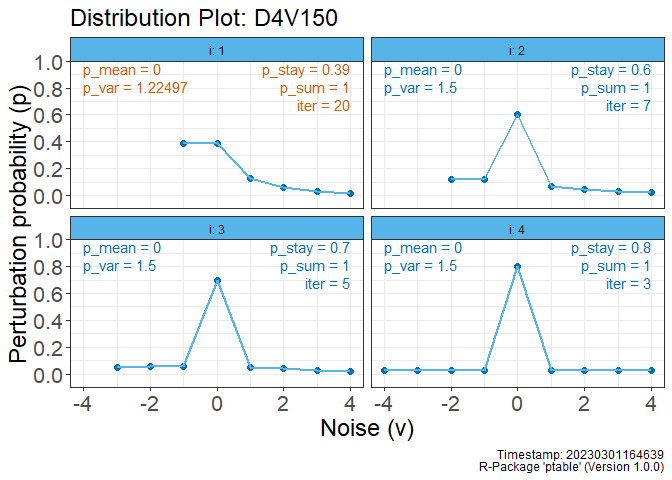Consequently, we would now have to adjust the variance to V=1.225 and re-run the computation:

result <-
create_cnt_ptable(D = 4,
V = 1.225,
pstay = c(0.8, 0.9, 0.9, 0.9))
plot(result, type = "d")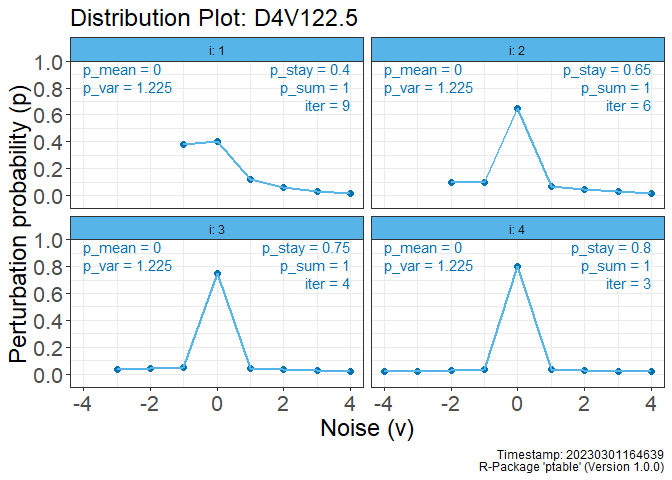Alternatively, we can change the monotony condition for i=1:

result <- create_cnt_ptable(
D = 4,
V = 1.5,
pstay = c(0.8, 0.9, 0.9, 0.9),
mono = c(FALSE, TRUE, TRUE, TRUE)
)
plot(result, type = "d")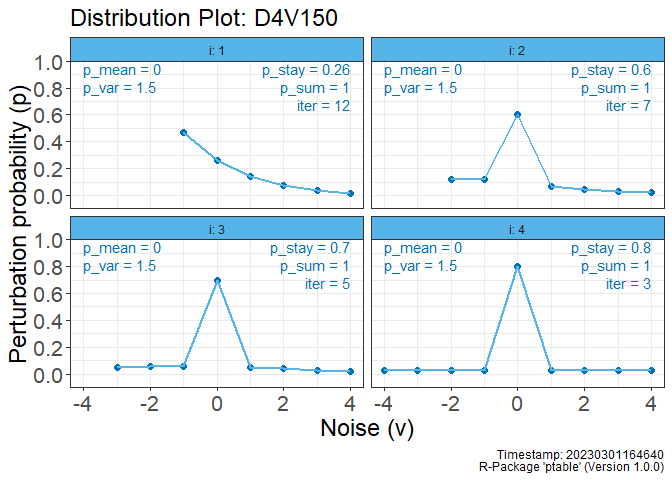# Magnitude Tables

## Simple Setting

### Setting the parameters for magnitude tables

If the goal is to create perturbation parameters suitable for numerical variables (magnitude tables), one may use function create_num_ptable() as shown below.

res <- create_num_ptable(D = 2, V = 1, icat = c(1, 2))

However, when creating objects for magnitude tables, users can also set the following arguments:

• icat (mandatory): The categories for the interpolation.
• type (optional): Distinction between odd and even numbers (see Giessing/Tent 2019).

1. In case of extended parameter settings, i.e. setting the argument pstay=... the algorithm tries to reduce the preset pstay-value iteratively in order to fulfill the fixed variance condition.↩︎

2. If false positives are an important part of a protection concept, we recommend to use (targeted record swapping)[https://github.com/sdcTools/CensusProtection/] as protection method (alone, or in combination with CKM, as proposed by the SGA “Harmonized protection of census data in the ESS”.↩︎